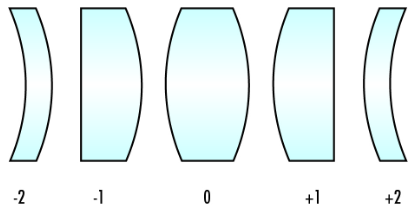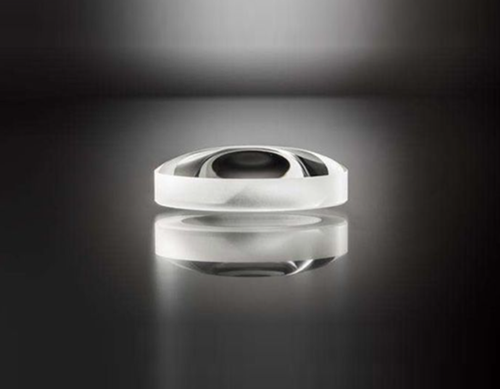# Shape Factor Influence in Aspheric Lens Design

Jan. 13, 2023

As the availability of aspheric lenses increases, optical system integrators should possess a basic knowledge of the design process to obtain the lens best suited for their needs. An asphere is an optical lens that does not have a constant radius of curvature, thus it is not a portion of a sphere. Aspheric lenses improve performance and can reduce the number of lens elements needed in several applications including laser-enabled devices, cinematography lenses, smartphone cameras, and surgical instruments.

When designing an aspheric lens, it is important to consider the application for which the specific lens is intended, as this will determine the shape factor of the lens. The shape factor of an asphere is a ratio of surface curvatures that describe the shape of the lens and typically ranges from values of -2 to +2 as shown in Figure 1. The sign of the shape factor is based upon the orientation of the lens with respect to the object being imaged. For example, a shape factor of +1 denotes that the convex, or curved, side of the lens faces the object.Figure 1: Examples of lenses with varying shape factors.

## Best Form Aspheric Lenses

Best form aspheric lenses are closed-form solutions, meaning that there is an explicit superior practice for designing these aspheres. While this makes them simpler to design, it also limits their abilities. The curved surface of best form aspheres begins with a base conic constant of -1. The conic constant is found using the equation $\small{ k = -1 \times n^2}$ where "$\small{n}$" is the refractive index of the glass or other material used for the lens.

Best form aspheric lenses achieve diffraction-limited focused spots on-axis for a given wavelength, but changing wavelengths result in chromatic aberrations and reduced performance for wavelengths different than the design wavelength. The lens's refractive index and spherical aberration correction vary with wavelength, which is known as spherochromatism. This makes best form aspheres ideal for monochromatic and highly-collimated applications, such as laser systems.

However, the flat surface of the asphere reflects incident light directly back to the source, which could be a cause for concern in certain laser applications. Using an asphere designed for a -1 configuration in a +1 configuration with the collimated source incident on the convex surface will result in poor performance.

## Prime Aspheric Lenses

Prime aspheric lens designs begin with a base conic of +1 and do not have closed-form solutions. Therefore, the design process is more involved and the even sphere asphere polynomial must be used to determine the surface sag of the desired lens.$$Z\left(s\right)= \frac {Cs^2}{1+\sqrt{1-\left(1+k\right)C^2s^2}}+A_4 s^4+A_6 s^6+A_8 s^8 + \, ...$$

Where:

$Z$: sag of surface parallel to the optical axis

$s$: radial distance from the optical axis

$C$: curvature, inverse of radius

$k$: conic constant

$A_4, \, A_6, \, A_8 \, ...$: 4th, 6th, 8th… order aspheric coefficients

The +1-shape factor of prime lenses denotes the orientation where the convex surface faces the object and in which both surfaces have power to refract incoming rays. While this configuration may be more effective in certain applications, it makes the design process more complex. For prime aspheres, a complication arises for lenses that have f/#’s approaching 1. (For more information on f/#, please see our System Throughput, f/#, and Numerical Aperture application note). When the curvature of a low f/# lens approaches that of a hemisphere, incoming light rays may be refracted at angles such that total internal reflection (TIR) occurs. This is mitigated by adding an arbitrary conic to the lens configuration so that the oblique rays may be corrected to be less than the critical angle.

In the polynomial above, the second and fourth terms are often adjusted to zero in asphere applications, as the second term affects the radius of the lens and the fourth term affects the conic constant. It is important to note that these variable constants depend on the optimization of the lens and the existence of a diffraction-limited spot size. In the case of prime aspheric lenses, the fourth term may vary as it will not affect optimization. Any inflection points in the design could increase the complexity and cost of manufacturing the lens.

## Comparison of Best Form and Prime Aspheric Lenses

While prime lenses require more time and involvement to design, their advantages over best form lenses are often well worth it. Best form lenses often struggle with spherochromatism and narrow field, as previously mentioned. Prime design allows for the mitigation of these difficulties. The orientation of prime aspheres allows for smaller spot sizes due to the refracting power being split between two surfaces instead of one. Additionally, this orientation causes prime aspheres to be less sensitive to changes in light wavelength. Finally, prime aspheres are better at handling off-axis rays and misalignment in systems. Off-axis performance is better suited to these lenses than best form designs. However, for all the advantages of prime aspheric lenses, they do have their disadvantages. When their orientation is flipped, performance will deteriorate, and total internal reflection may occur. Thankfully, this is easily avoided by being familiar with the intended design of prime aspheres and recognizing their correct orientation.

Overall, the most important factor in aspheric lens design is open and thorough communication between the designer and manufacturer. This allows for the shape factor and other lens components to be carefully considered so that errors are avoided, and the correct lenses are implemented for every application. Contact us to discuss your application and ensure that you leverage the right optical components to achieve your application goals.